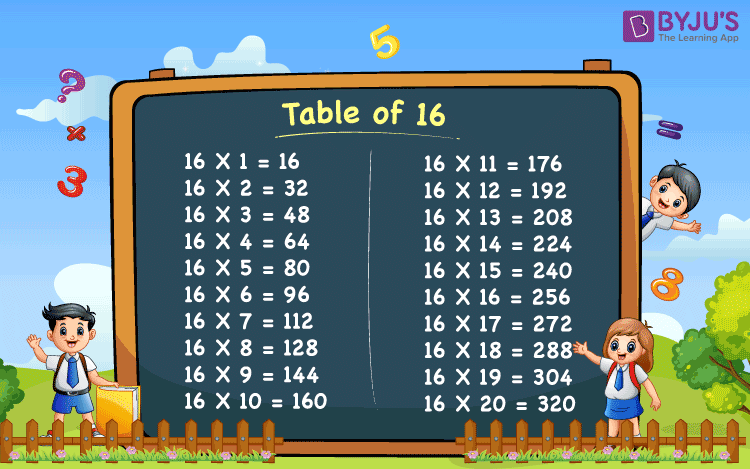# Table of 16

Table of 16 is the multiplication table that includes the multiples of 16.  Students studying in class 9 onwards should try to memorize the multiplication table until 20. Memorizing the multiplication table will help them in saving time while solving complicated mathematical problems. Students who are preparing for competitive exams should also try to memorize the multiplication table of 16 or 16 table for faster calculation during the exam. To help the students, we have provided the 16 times table.

Students can also download the table of 16 PDF from below and learn offline as well.

## Table of 16 Chart

Primary class students would find this 16 table chart interesting to read and learn.## Multiplication Table of 16

The table of 16 or 16 times table results in multiples of the number 16 when it is multiplied by a set of whole numbers.  Here is the 16 table for students up to 20 times, which can help them with multiplication problems.

Table of 16 is formed by repeatedly adding 16 to itself. Such as:

16+16 = 32

16+16+16 = 48

16+16+16+16 = 64

And so on.

## 16 Times Table

Go through the below 16 table or 16 times table and solve the problems quickly by learning them.

 16 × 1 = 16 16 × 2 = 32 16 × 3 = 48 16 × 4 = 64 16 × 5 = 80 16 × 6 = 96 16 × 7 = 112 16 × 8 = 128 16 × 9 = 144 16 × 10 = 160 16 × 11 = 176 16 × 12 = 192 16 × 13 = 208 16 × 14 = 224 16 × 15 = 240 16 × 16 = 256 16 × 17 = 272 16 × 18 = 288 16 × 19 = 304 16 × 20 = 320

### Tips to Memorize 16 Table in Maths

Students may find learning the 16 table little challenging. Hence, here are a few tips and tricks to learn the table of 16 for students, such that it can be easily memorised.

1. The pattern of the table of 16 is such that, the digits at the unit place are followed by 6, 2, 8, 4, 0 and then repeated.
2. All the multiples of 16 are even numbers
3. 16 times of any even number, will have the same digit at the unit place as the even number (16 x 2 = 32, 16 x 4 = 64, etc.)
4. You can skip read table of 8 for odd numbers to get 16 times table, such as, 8 x 2 = 16, 8 x 4 = 32, 8 x 6 = 48, 8 x 8 = 64, 8 x 10 = 80 and so on. We can see, it gives a table of 16 in consecutive manner: 16, 32, 48, 64, 80

## Examples on 16 Table

Example 1:

What is the value of 16 times 8 plus 16 times 10?

Solution:

From the table of 16, we have;

16 times 8 = 16 x 8 = 128

16 times 10 = 16 x 10 = 160

Sum = 128 + 160 = 288

Example 2:

Find the difference: 16 x 11 – 100.

Solution:

From the table of 16, we have;

16 x 11 = 176

Thus, the required difference is:

176 – 100 = 76

Example 3:

Radha brought 5 packets of toffees. Each packet cost Rs.16. What is the total cost of packets?

Solution:

Given,

Number of packets of toffees = 5

Cost of one toffee packet = Rs.16

Total cost of 5 packets = Rs.16 x 5 = Rs.80

## Practice Questions

1. What is the value of 16 times 7?
2. Find the sum of 16 times 4 and 16 times 12.
3. What is the value of 16 times 3 plus 121?

## Frequently Asked Questions on Table of 16

Q1

### What is Table of 16?

Table of 16 when 16 is multiplied by 1 till 10 is given by:
16, 32, 48, 64, 80, 96, 112, 128, 144 and 160
Q2

### How do we get a table of 16?

Table of 16 provides the result when 16 is added repeatedly to itself.
16+16 = 32
16+16+16 = 48
16+16+16+16 = 64
16+16+16+16+16=80
And so on
Q3

### How do we read 16 times table?

16 ones are 16, 16 twos are 32, 16 threes are 48, 16 fours are 64, 16 fives are 80, 16 sixes are 96, 16 sevens are 112, 16 eights are 128, 16 nines are 144 and 16 tens are 160.
Q4

### What is 16 times 16?

16 times of sixteen is equal to 256.
Q5

### What is 20 times sixteen?

20 times of sixteen is 320.
Test your knowledge on Table Of 16